You are viewing documentation for an older version. For current documentation - click here.

Let us take a look at the Rectangle Annotation here. It is one of the simplest annotations you can have on the chart. A rectangle annotation will look as under: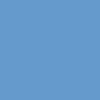The XML/JSON to create the above rectangle is:

```<chart>
...
<annotations>
<annotationGroup id="Grp1">
<annotation type="rectangle" color="639ACE" x="200" y="200" toX="300" toY="300"/>
</annotationGroup>
</annotations>
...
</chart>```
```{
"chart": {},
...
"annotations": {
"groups": [
{
"id": "Grp1",
"items": [
{
"type": "rectangle",
"color": "639ACE",
"x": "200",
"y": "200",
"tox": "300",
"toy": "300"
}
]
}
]
}
...
}```

As it is very clear from the code above, we need to set the annotation type to rectangle, specify the starting x and y co-ordinates and the ending x and y co-ordinates and also a color for the rectangle.

Now suppose we want the rectangle to have a gradient background.All we need to do is to change the code to the following.

```<chart>
...
<annotations>
<annotationGroup id='Grp1' >
<annotation type='rectangle' color='639ACE,FFFFFF' x='200' y='200' toX='300' toY='300'/>
</annotationGroup>
</annotations>
...
</chart>```
```{
"chart": {},
...
"annotations": {
"groups": [
{
"id": "Grp1",
"items": [
{
"type": "rectangle",
"color": "639ACE,FFFFFF",
"x": "200",
"y": "200",
"tox": "300",
"toy": "300"
}
]
}
]
...
}
}```

Thus, we have added FFFFFF to the color list to make a gradient. You can add as many colors as you want to this gradient color list. Let us add another color to the gradient - let's make the color attribute as color='639ACE,FFFFFF,FF5904':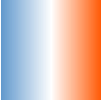Now let's change the gradient angle. The same can be done using fillAngle attribute. fillAngle='90' gives: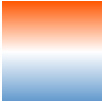We can also set the ratio in which the constituent colors of the gradient will be divided using fillRatio attribute. Since we have 3 colors in the gradient here, we need 3 values in fillRatio, which should sum up to 100. Setting fillRatio='20,10,70' will give:If you need to set the alpha (transparency) of the constituent colors of the gradient, then you have to use the fillAlpha attribute. Again this attribute should have as many values as there are colors in the gradient. For this gradient having 3 colors, one of the various combinations could be fillAlpha='50, 100, 50':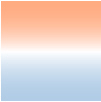All we need to do is add showBorder='1' to the <annotation> element. Now let's make the rectangle round-edged.To achieve the above, we need to add radius='10' to the <annotation> element. The border color and thickness can be customized using the borderColor and borderThickness attributes. Setting borderColor='333333' and borderThickness='4' will give: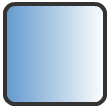Thus our final XML/JSON will look as under:

```<chart>
...
<annotations>
<annotationGroup id='Grp1' >
<annotation type='rectangle' color='639ACE,FFFFFF' x='200' y='200' toX='300' toY='300' showBorder='1'
</annotationGroup>
</annotations>
...
</chart>```
```{
"chart": {},
...
"annotations": {
"groups": [
{
"id": "Grp1",
"items": [
{
"type": "rectangle",
"color": "639ACE,FFFFFF",
"x": "200",
"y": "200",
"tox": "300",
"toy": "300",
"showborder": "1",
"bordercolor": "333333",
"borderthickness": "4"
}
]
}
]
...
}
}```

Thus, using the various attributes for the rectangle annotation type, you can customize it as per your requirements.

ApplicationThe XML for the above is:

```<chart ... >
...
<annotations>
<annotationGroup id='Grp1' showBelow='1'>
<annotation type='rectangle' x='0' y='0' toX='400' toY='200' radius='10' fillColor='333333, 453269'
fillAngle='180' />
<annotation type='rectangle' x='5' y='5' toX='395' toY='195' radius='10' color='000000' fillAlpha='0'
showBorder='1' borderColor='FFFFFF' borderThickness='3'/>
</annotationGroup>
</annotations>
</chart>```
```{
"chart": {...},
...
"annotations": {
"groups": [
{
"id": "Grp1",
"showbelow": "1",
"items": [
{
"type": "rectangle",
"x": "0",
"y": "0",
"tox": "400",
"toy": "200",
"fillcolor": "333333, 453269",
"fillangle": "180"
},
{
"type": "rectangle",
"x": "5",
"y": "5",
"tox": "395",
"toy": "195",